# Introduction to Quantum Computing

“I think I can safely say that nobody understands quantum mechanics.” ~ Richard Feynman.

# Hilbert space

Hilbert space is generalization of the Euclidean space. In a Hilbert space we can have infinite number of dimensions.

A vector in an infinite-dimensional Hilbert space is represented as an infinite vector: $$(x_1, x_2, ….)$$.

The inner product of two vectors $$?x|y? = \sum_{i=1}^? x_i y_i$$

The norm $$||x|| = ?x|x?^{\frac{1}{2}} = \sqrt{\sum_{i=1}^? x_i^2} < ?$$

Tensor product of two vectors $$\begin{bmatrix} a_1 \\ a_2 \end{bmatrix} ? \begin{bmatrix} b_1 \\ b_2 \end{bmatrix} = \begin{bmatrix} a_1 * b_1 \\ a_1 * b_2 \\ a_2 * b_1 \\ a_2 * b_2 \end{bmatrix}$$

# Qubit

In quantum computing, a qubit or quantum bit is a unit of quantum information. It’s the analog of a bit for quantum computation. A qubit is a two-state quantum-mechanical system. The state 0 could be represented as vector $$\begin{bmatrix} 1 \\ 0 \end{bmatrix} ? |0? ? |??$$ (horizontal polarization) and state 1 could be represented as orthogonal vector $$\begin{bmatrix} 0 \\ 1 \end{bmatrix} ? |1? ? |??$$ (vertical polarization) .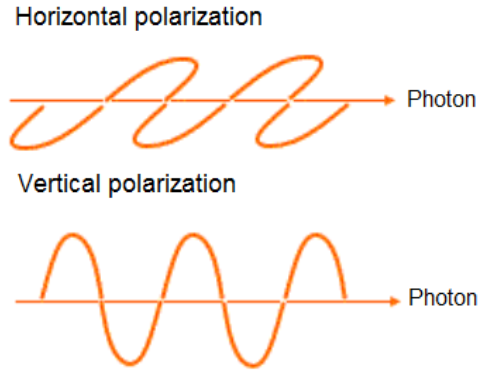A generic qubit state corresponds to a vector $$\begin{bmatrix} ? \\ ? \end{bmatrix} = ? \begin{bmatrix} 1 \\ 0 \end{bmatrix} + ? \begin{bmatrix} 0 \\ 1 \end{bmatrix} ? ? \ |0? + ? \ |1?$$ in a two-dimensional Hilbert space such as $$|?|^2 + |?|^2 = 1$$ (normalized vector). $$|?|^2, |?|^2$$ are the probabilities for the particle to be in state 0 and 1. ? and ? are complex numbers and they are called amplitudes.

Another way to write the state ? of a qubit is by using cos and sin functions.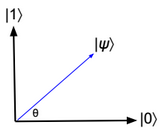|?? = cos(?) |0? + sin(?) |1?

More details about qubit can be found here: https://arxiv.org/pdf/1312.1463.pdf

# Two qubits

A state in a combined two qubits system corresponds to a vector in four-dimensional Hilbert space $$|\gamma? = e \ |00? + f \ |01? + g \ |10?+ h \ |11? = \begin{bmatrix} e \\ f \\ g \\ h \end{bmatrix}$$.

In general, the state vector of two combined systems is the tensor product of the state vector of each system.

Some expressions and their equivalents:

|0??|0??|0? ? |000?

|0??|1??|0? ? |010?

$$(\sqrt{\frac{1}{2}}|0? + \sqrt{\frac{1}{2}}|1?)?(\sqrt{\frac{1}{2}}|0? + \sqrt{\frac{1}{2}}|1?) ? \frac{1}{2}|00? + \frac{1}{2}|01? + \frac{1}{2}|10? + \frac{1}{2}|11?$$

# Bloch sphere

A state ? of a qubit can be also written as: $$|?? = cos(\frac{?}{2})|0? + sin(\frac{?}{2}) exp(i?) |1?$$, and it can be visualized as a vector of length 1 on the Bloch sphere.

The angles ?,? correspond to the polar and azimuthal angles of spherical coordinates (??[0,?], ??[0,2?]).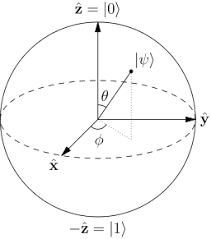# Bra-ket notation

|01? ? |0??|1? ? $$\begin{bmatrix} 0 \\ 1 \\ 0 \\ 0 \end{bmatrix}$$ (column vector)

?01| ? ?0|??1| ? $$\begin{bmatrix} 0 \ 1 \ 0 \ 0 \end{bmatrix}$$ (row vector)

# Quantum superposition

An arbitrary state of a qubit could be described as a superposition of 0 and 1 states.

# N qubits

A 3 qubits quantum system can store numbers such as 3 or 7.

|011? ? |3?

|111? ? |7?

But it can also store the two numbers simultaneously using a superposition state on the last qubit.

$$(\sqrt{\frac{1}{2}}|0? + \sqrt{\frac{1}{2}}|1?)?|1??|1? ? \sqrt{\frac{1}{2}} (|011? + |111?)$$

In general, a quantum computer with n qubits can be in an arbitrary superposition of up to $$2^n$$ different states simultaneously.

# Quantum gates

The Hadamard gate acts on a single qubit. It maps the state |0? to a superposition state with equal probabilities on state 0 and 1 ($$\sqrt{\frac{1}{2}}|0? + \sqrt{\frac{1}{2}}|1?$$).

The Hadamard matrix is defined as: $$H = \frac {1}{\sqrt {2}} \begin{bmatrix}1 \ 1 \\ 1 \ -1 \end{bmatrix}$$

$$H * \begin{bmatrix} 1 \\ 0 \end{bmatrix} = \begin{bmatrix} \sqrt{\frac{1}{2}} \\ \sqrt{\frac{1}{2}} \end{bmatrix}$$

Pauli X gate (NOT gate)

The Pauli-X gate acts on a single qubit. It is the quantum equivalent of the NOT gate for classical computers.

The Pauli X matrix is defined as: $$X = \begin{bmatrix}0 \ 1 \\ 1 \ 0 \end{bmatrix}$$

$$X * \begin{bmatrix} 1 \\ 0 \end{bmatrix} = \begin{bmatrix} 0 \\ 1 \end{bmatrix}$$

Pauli Y gate

The Pauli-Y gate acts on a single qubit. It equates to a rotation around the Y-axis of the Bloch sphere by ? radians. It maps |0? to i |1? and |1? to ?i |0?

The Pauli Y matrix is defined as: $$Y = \begin{bmatrix}0 \ -i \\ i \ 0 \end{bmatrix}$$

$$Y * \begin{bmatrix} 1 \\ 0 \end{bmatrix} = \begin{bmatrix} 0 \\ i \end{bmatrix}$$

Pauli Z gate

The Pauli-Z gate acts on a single qubit. It equates to a rotation around the Z-axis of the Bloch sphere by ? radians. It leaves the basis state |0? and maps |1? to ?|1?

The Pauli Z matrix is defined as: $$Z = \begin{bmatrix}1 \ 0 \\ 0 \ -1 \end{bmatrix}$$

$$Z * \begin{bmatrix} 1 \\ 0 \end{bmatrix} = \begin{bmatrix} 1 \\ 0 \end{bmatrix}$$

Other gates are described in this link: https://en.wikipedia.org/wiki/Quantum_gate

# State measurement

State measurement can be done using polarizing filters. Filters transmit or block particles based on angle of polarization.

# IBM Q

IBM Q is a cloud quantum computing platform.

Below a simple experiment where we have two qubits. No transformation is applied on first qubit. A Pauli X transformation is applied on second qubit. As result there is one possible state |10? with probability equals 1.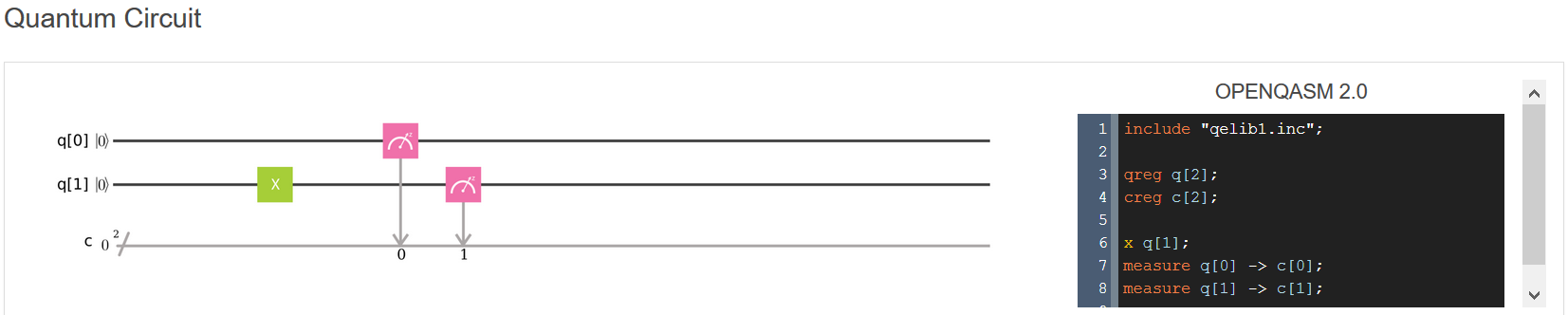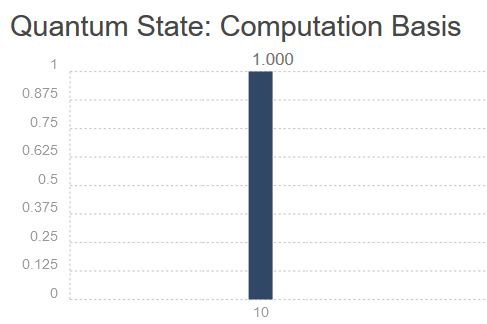# Data representation

Given a vector $$x = (x_1, x_2, …. , x_n)$$. The quantum system topology needed to store the vector x is $$log_2(n)$$ qubits.

For example, the quantum system representation of the following vector requires only 3 qubits.

(0, 0.2, 0.2, 0, 0.1, 0.2, 0.1, 0.3) ? 0 |000? + 0.2 |001? + 0.2 |010? + 0 |011? + 0.1 |100? + 0.2 |101? + 0.1 |110? + 0.3 |111?